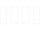На главную

# The differential and integral calculus : containing differentiation, integration, development series, differential equations, differences, summation, equations of differences, calculus of variations, definite integrals, with applications to algebra, plane geometry, solid geometry, and mechanics. Also, : Elementary ill. of the differential and integral calculus

The differential and integral calculus : containing differentiation, integration, development series, differential equations, differences, summation, equations of differences, calculus of variations, definite integrals, with applications to algebra, plane geometry, solid geometry, and mechanics. Also, : Elementary ill. of the differential and integral calculus

XX, 786, 64,  с.

Российская Национальная Библиотека

Полное библиографическое описание

• 100% от первой страницы документа

• Произвольный отрывок, составляющий 25% документа
• 100% от первой страницы документа

• Произвольный отрывок, составляющий 25% документа
• 100% от первой страницы документаОткрыть в мобильном приложении